## 一、箍筋按外皮长度计算

（一）箍筋按外皮计算的演变过程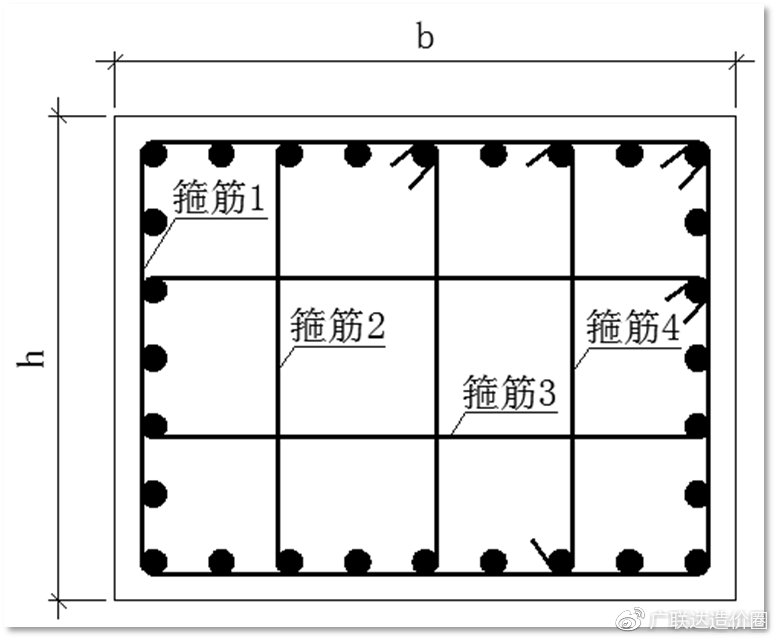图1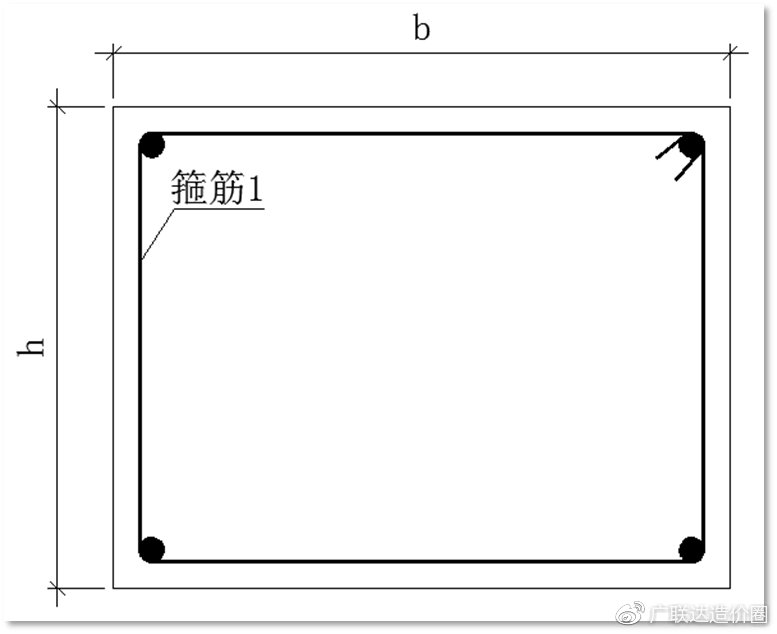图2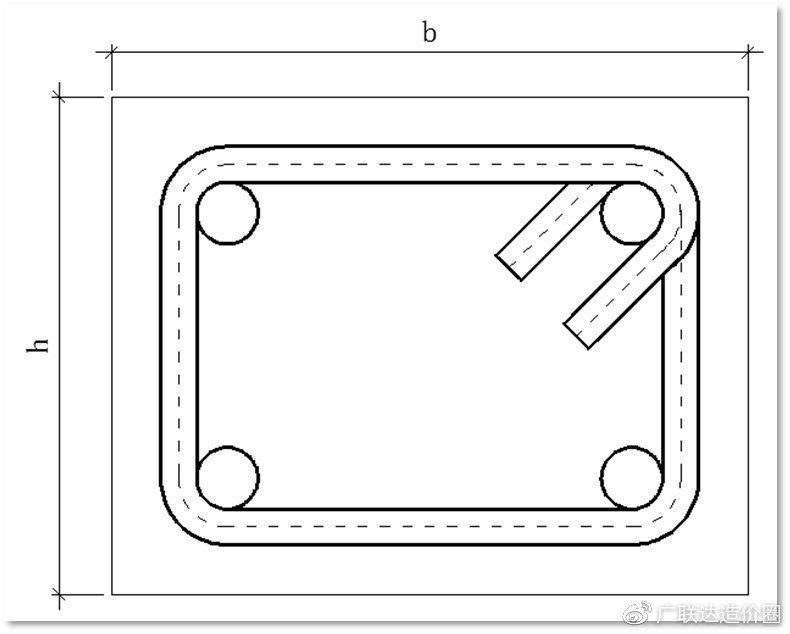图3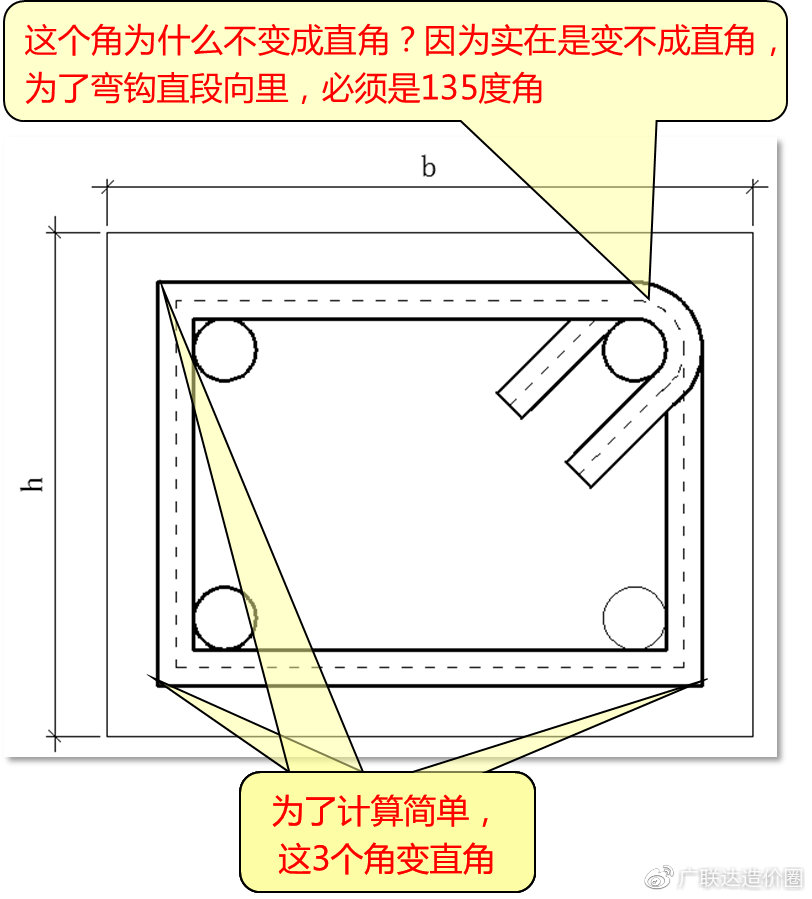图4

（二）箍筋按外皮计算公式推导过程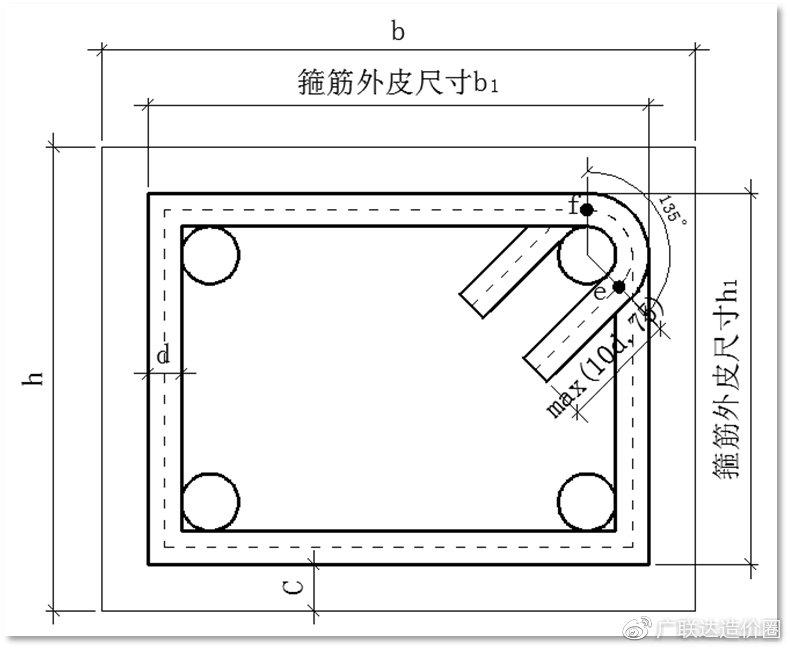图5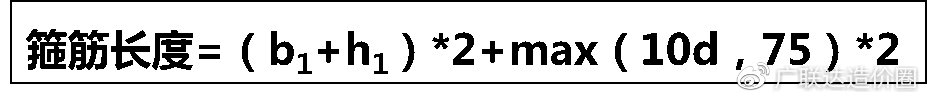图6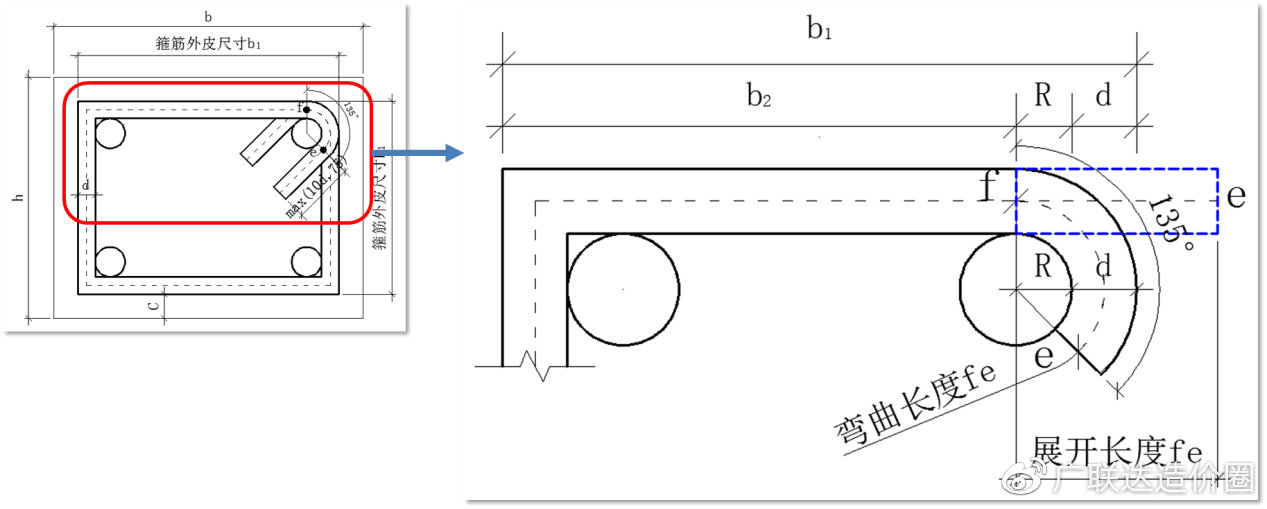图7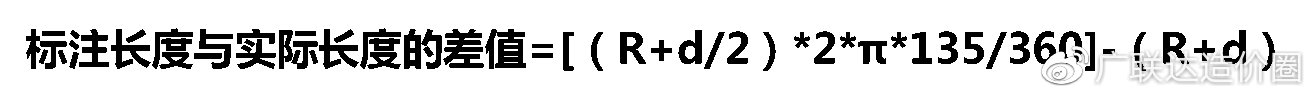图8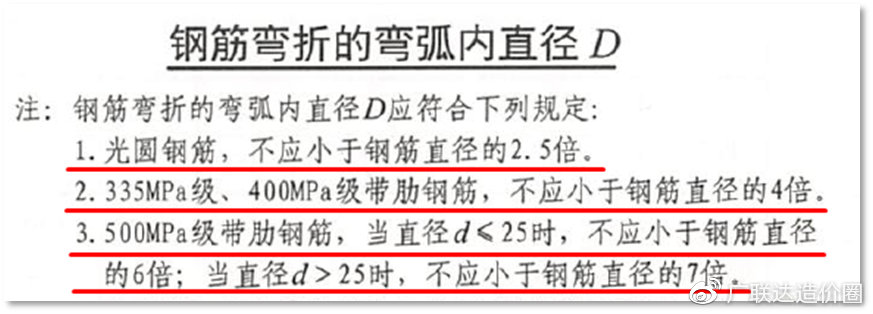图9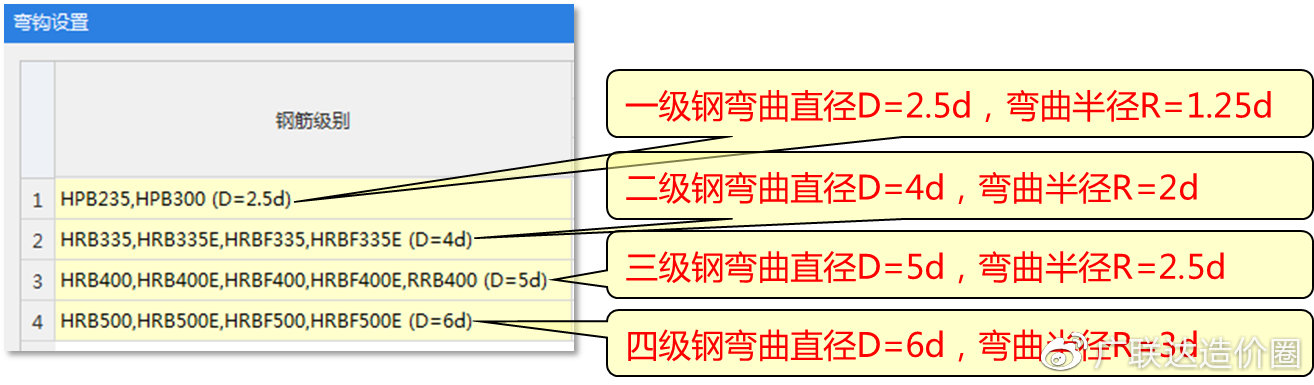图10图11

1、当弯曲半径=1.25d时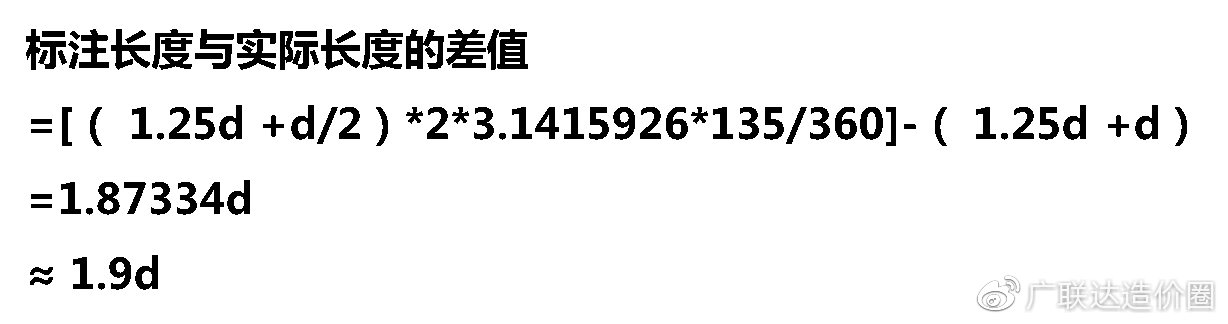2、当弯曲半径=2d时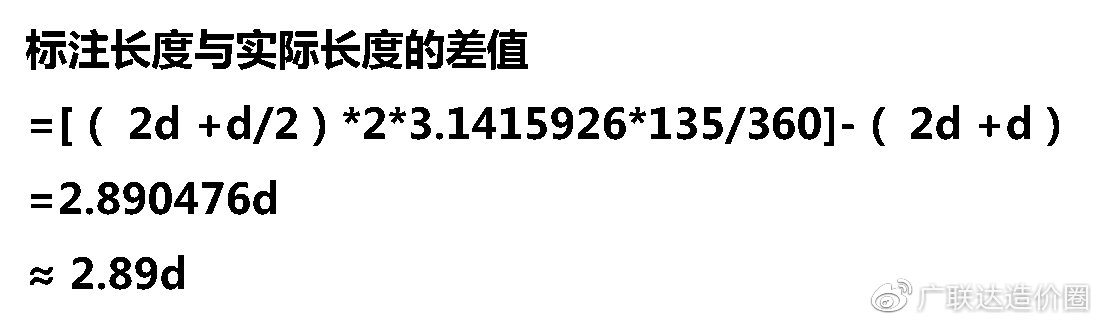3、当弯曲半径=2.5d时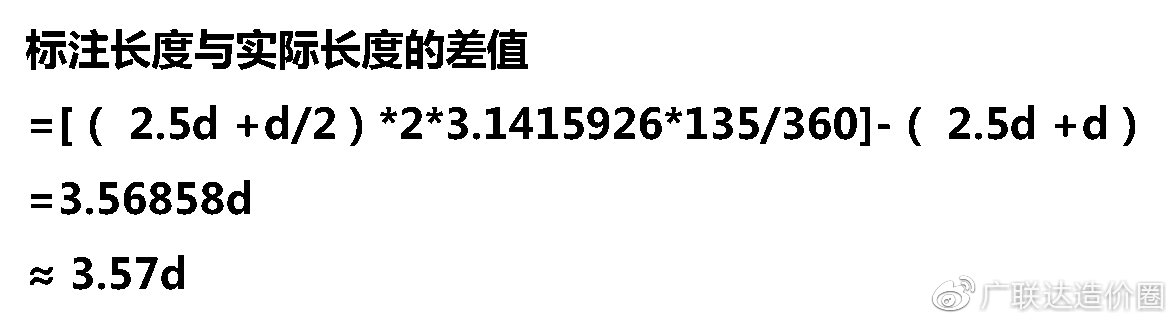4、当弯曲半径=3d时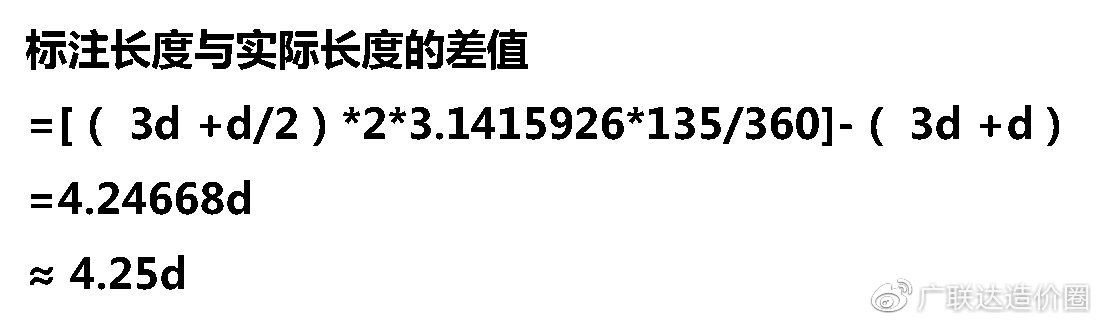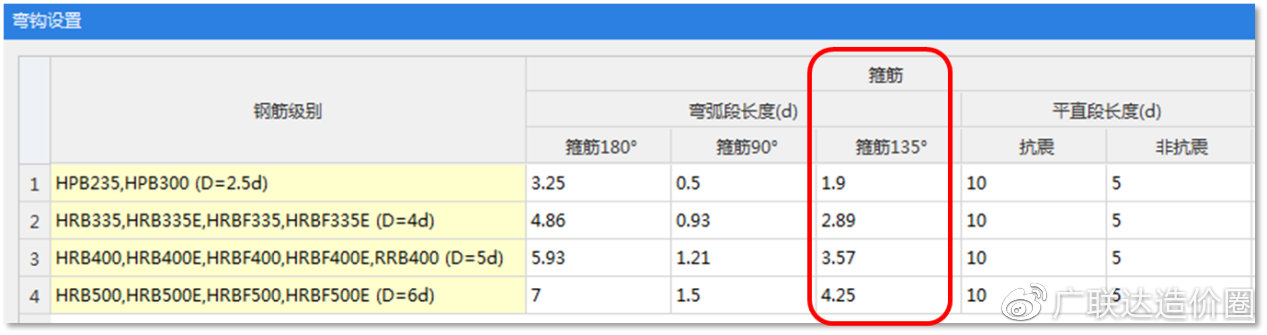图12

5、按外皮计算公式推导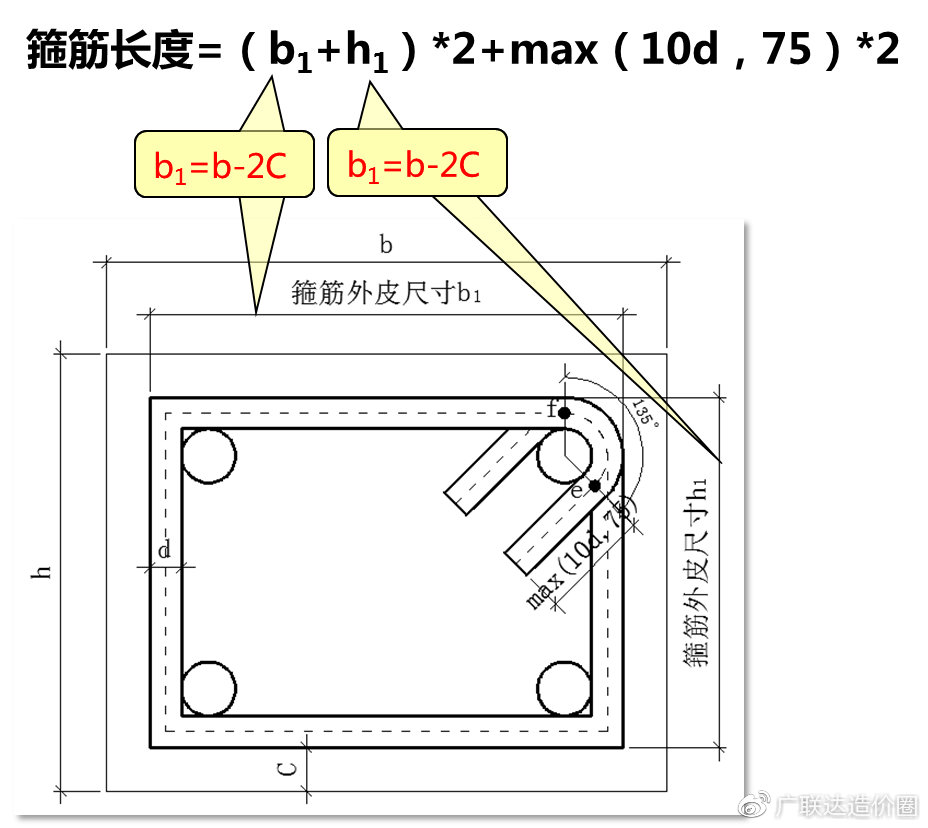图13图14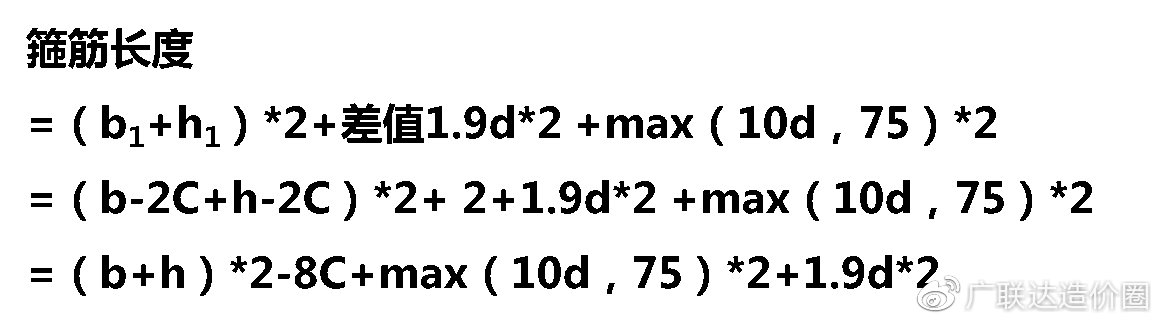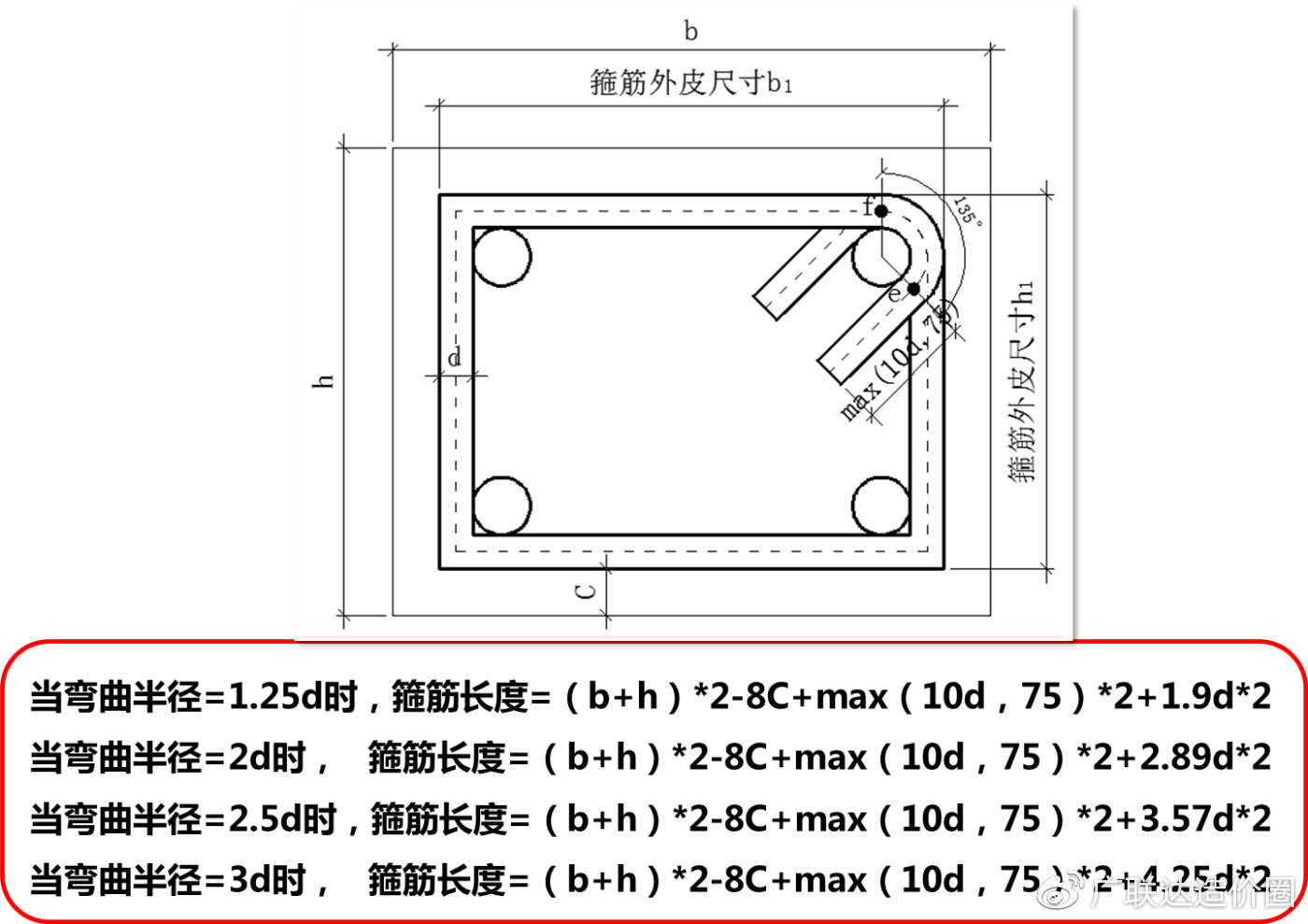图15

## 二、箍筋按中心线长度计算公式推导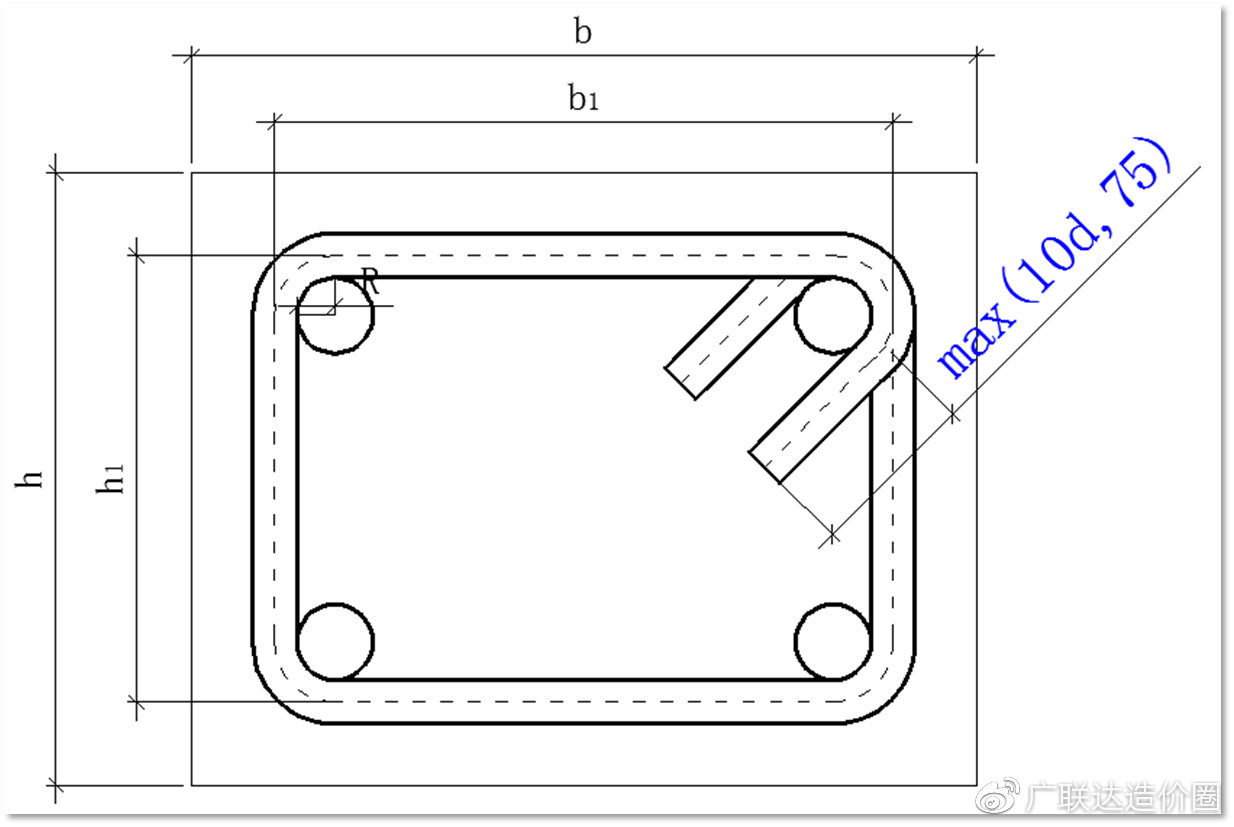图16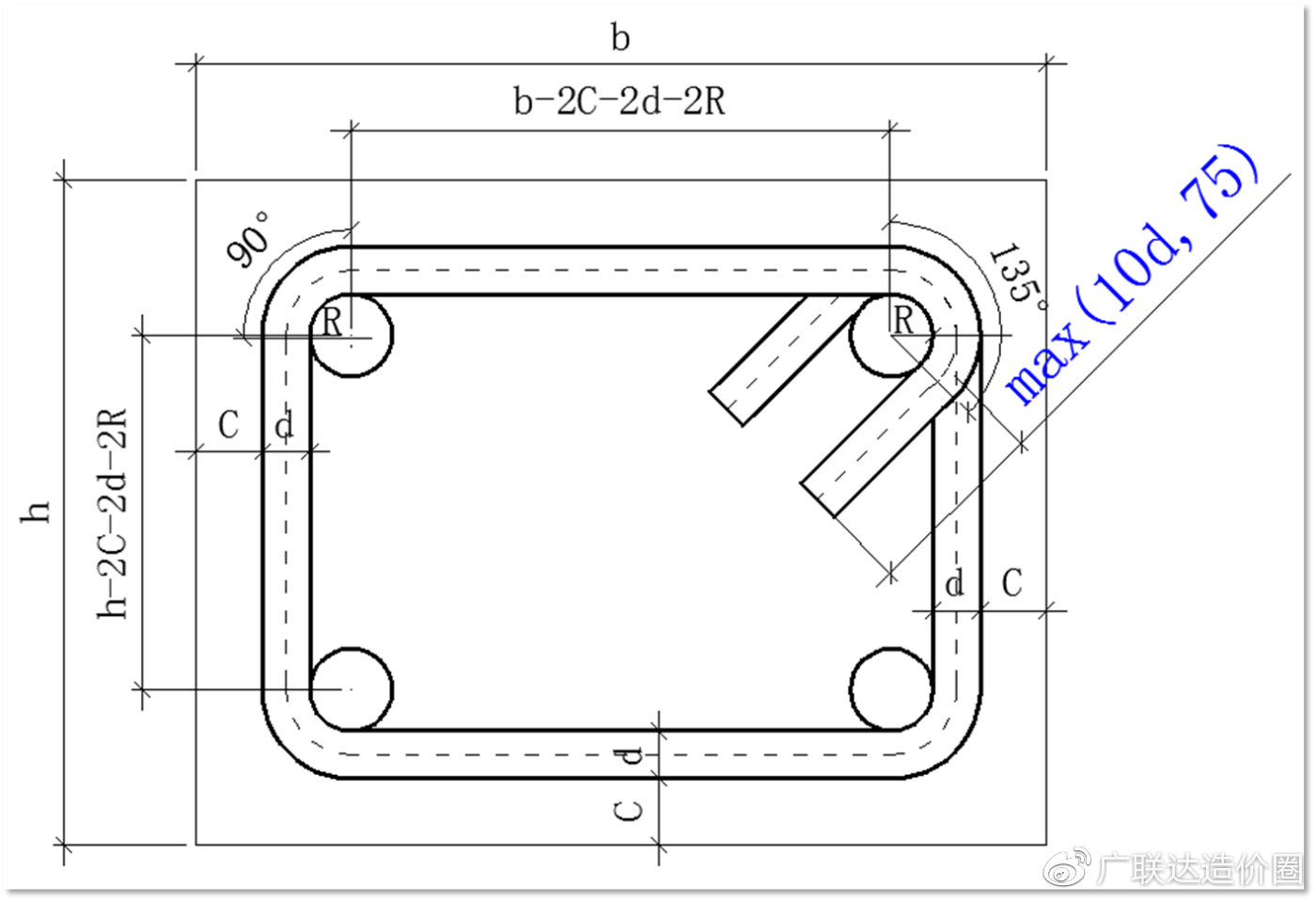图17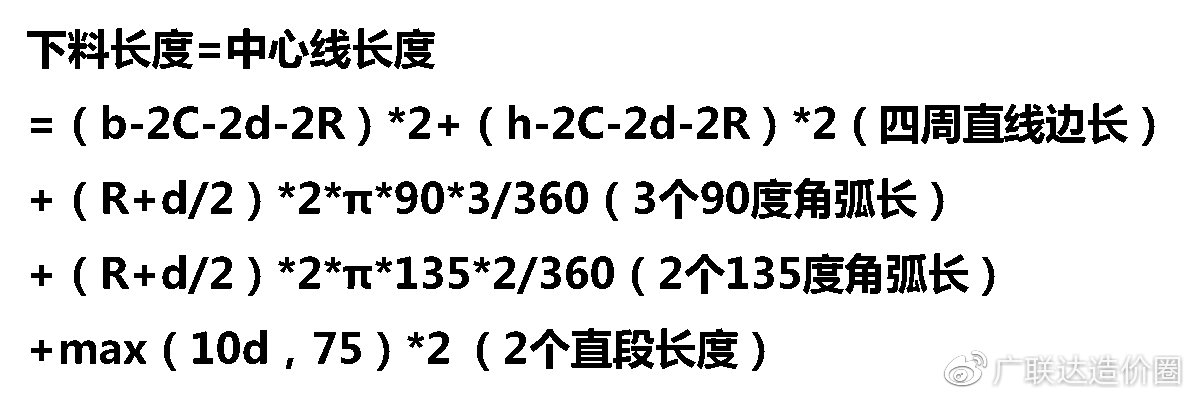图18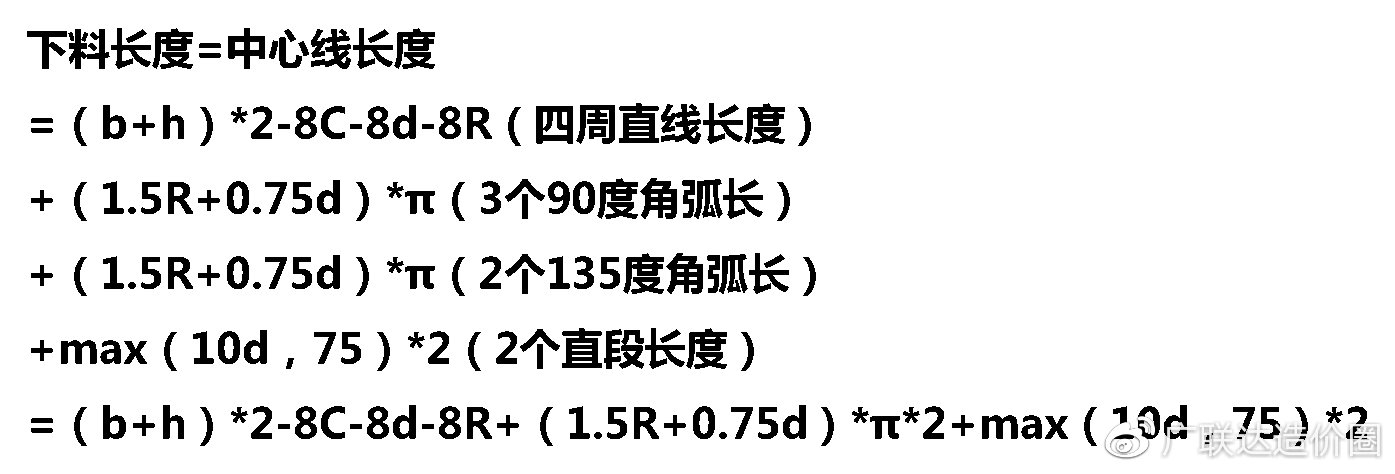图19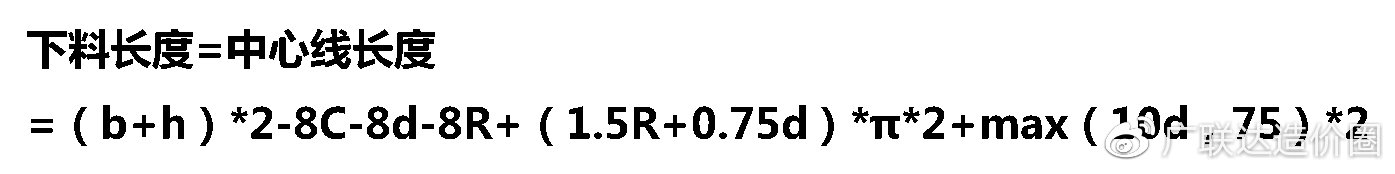图20

## 三、箍筋按外皮计算与按中心线计算长度比较

（一）当箍筋是一级圆钢时，弯曲半径R=1.25d

1、箍筋按外皮计算长度

=（b+h）*2-8C+max（10d，75）*2+1.9d*2

=（500+500）*2-8*30+80*2+1.9*8*2

=1950.4

2、箍筋按中心线计算长度

=(b+h)*2-8C-8d-8R+(1.5R+0.75d)*π*2

+max(10d，75)*2

=(500+500)*2-8*30-8*8-8*1.25*8

+(1.5*1.25*8+0.75*8)*3.1415926*2

+80*2=1907.95

3、二者差值

（二）当箍筋是二级螺纹钢时，弯曲半径R=2d

1、箍筋按外皮计算长度

=（b+h）*2-8C+max（10d，75）*2+1.9d*2

=（500+500）*2-8*30+80*2+2.89*8*2

=1966.24

2、箍筋按中心线计算长度

=（b+h）*2-8C-8d-8R+（1.5R+0.75d）

*π*2+max（10d，75）*2

=（500+500）*2-8*30-8*8-8*2*8

+(1.5*2*8+0.75*8)*3.1415926*2+80*2

=1916.50

3、二者差值

（三）当箍筋是三级螺纹钢时，弯曲半径R=2.5d

1、箍筋按外皮计算长度

=（b+h）*2-8C+max（10d，75）*2+1.9d*2

=（500+500）*2-8*30+80*2+3.57*8*2

=1977.12

2、箍筋按中心线计算长度

=(b+h)*2-8C-8d-8R+(1.5R+0.75d)*π*2

+max(10d,75)*2

=(500+500)*2-8*30-8*8-8*2.5*8

+(1.5*2.5*8+0.75*8)*3.1415926*2

+80*2=1922.19

3、二者差值

（四）当箍筋是四级螺纹钢时，弯曲半径R=3d

1、箍筋按外皮计算长度

=（b+h）*2-8C+max（10d，75）*2+1.9d*2

=（500+500）*2-8*30+80*2+4.25*8*2

=1988

2、箍筋按中心线计算长度

=（b+h）*2-8C-8d-8R+（1.5R+0.75d）*π*2

+max（10d，75）*2

=（500+500）*2-8*30-8*8-8*3*8

+（1.5*3*8+0.75*8）*3.1415926*2+80*2

=1927.89

3、二者差值

## 四、实际工程中到底按哪个长度计算

1、如果当地定额有明确的规定，那就按定额规定计算；

2、如果当地定额没有规定，张向荣老师之前的工程中是按外皮长度计算的，个人经验，仅供参考。

#你的计算原则是什么？欢迎留言交流！#

*本文系张向荣原创，独家来稿(作者) 内容检查中 未能发表

@

28条评论

@60万造价人学习/交流的行业平台